# Heart rate analysis in normal subjects of various age groups

## Abstract

### Background

Analysis of heart rate variation (HRV) has become a popular noninvasive tool for assessing the activities of the autonomic nervous system (ANS). HRV analysis is based on the concept that fast fluctuations may specifically reflect changes of sympathetic and vagal activity. It shows that the structure generating the signal is not simply linear, but also involves nonlinear contributions. Linear parameters, Power spectral indice (LF/HF) is calculated with nonlinear indices Poincare plot geometry(SD1,SD2), Approximate Entropy (ApEn), Largest Lyapunov Exponent (LLE) and Detrended Fluctuation Analysis(DFA). The results show that, with aging the heart rate variability decreases. In this work, the ranges of all linear and nonlinear parameters for four age group normal subjects are presented with an accuracy of more than 89%.

As a pre-analysis step, the HRV data is tested for nonlinearity using surrogate data analysis and the results exhibited a significant difference in the ApEn, LLE, SD1/SD2 and DFA parameters of the actual data and the surrogate data.

### Methods

The heart rate is analyzed using the various time domain parameters, frequency domain parameter and nonlinear parameters like Poincare geometry, ApEn, LLE and DFA.

### Results

In this work, the different linear and nonlinear parameters evaluated show a particular range for various cardiac abnormalities. And the results of these were subjected to 't' test with more than 89% confidence interval giving excellent 'p' values in all cases.

### Conclusions

Heart rate variability (HRV) signal can be used as a reliable indicator of state of the heart. It becomes less random with the aging(less chaotic). This is evaluated by using various time domain, frequency domain and nonlinear parameters like SD1/SD2, ApEn, LLE α s and α l . Different ranges of non-linear parameters for various age groups are presented with 'p' value ≤ 0.12.

## Background

The Heart rate variability (HRV) is a non-invasive index of the neural control of the heart. HRV can be quantified by the simple calculation of the standard deviation of the R-R intervals. Furthermore, in the frequency domain, spectral analysis of HRV reveals three distinct frequency regions in the modulation of heart rate in humans. The typical spectral pattern, in normal conditions shows the presence of three frequency bands: a very low frequency (VLF) band from 0.00 to 0.03 Hz, a low frequency (LF) band from 0.03 to 0.15 Hz and a high frequency (HF) band in respiratory range generally more than 0.15 Hz. The power of LF component seems to be related to the vagal and sympathetic activities (LF component increases with every form of sympathetic stimulation), whereas the area of high frequency component (HF) provides a quantitative index of the influence of respiration on ECG signal and may be connected to the vagal activity. Thus LF/HF ratio is an important marker of sympathetic modulation or sympatho-vagal balance on heart rate variability control.

Physiological signals often vary in a complex and irregular manner. Analysis of linear statistics such as mean values, variability measures, and spectra of such signals generally does not address directly their complexity and thus may miss potentially useful information. Since the underlying mechanisms involved in the control of heart rate is mainly nonlinear, the application of nonlinear techniques seems appropriate [17, 14, 7, 2].

Recently, new dynamic methods of HRV quantification have been used to uncover nonlinear fluctuations in heart rate that are not otherwise apparent. Several methods have been proposed: Lyapunov exponents , 1/f slope , approximate entropy(ApEn)  and detrended fluctuation analysis.

Compared to men, women are at lower risk of coronary heart disease  and of serious arrhythmias , suggesting a beneficial difference in autonomic control of heart rate.

Previous studies have assessed gender and age-related differences in time and frequency domain indices  and some nonlinear component of HRV . There also seemed to be a significant difference between day and night hours when studying HRV indices using spectral and time domain methods [27, 37].

Terry et al, have shown that the middle-aged women and men have a dominant parasympathetic and sympathetic regulation of the heart rate, respectively .

It is proved that, the heart rate variability depends on the sex also. The heart rate variability is more in the physically active young and old women [29, 15]. The reduced HRV results in new cardiac events are studied by Hisako et al. It is proved by Emese et al, that the alert new borns heart rate variation is lower in the case of boys than in the case of girls . The Heart rate variation for healthy subjects from 20–70 yrs is studied by Hendrik et al, and found that the HRV decreases with age and variation is more in the case of female than men . Galeev et al, have analyzed the heart rate variation for healthy subjects of age from 6 to 16 yrs and observed the statistical and frequency domain variation with aging and gender .

In this work, we have analyzed the heart rate variation of normal subjects from 5–70 yrs in four groups (10 ± 5, 25 ± 5, 45 ± 5, 65 ± 5) by statistical method, frequency domain method and nonlinear method.

## Materials and Method

In this project, ECGs are recorded from 150 healthy Chinese origin subjects (75 male and 75 female) with age ranging from 5 to 70 years for 20 minutes in lead II configuration. First the data is recorded in the relaxed sitting position. The total ECG data recorded is divided into four groups: little children, young, middle aged and old subjects. The number of subjects in each group is shown in table 1. Later for the same duration ECG is recorded with the same subject in the lying position. The ECG is recorded using the PowerLab/16SP system (ADI instrument). The data is sampled at a sampling rate of 400 Hz and stored in a random access file. The interval between two successive QRS complexes is defined as the r-r interval (tr-r seconds)  and the heart rate (beats per minute) is given as:

HR = 60/tr-r     (1)

Table 1: Number of subjects in various groups

### Analysis

The heart rate is analyzed in the time domain, frequency domain and by using nonlinear parameters.

### Time-and Frequency-Domain Analysis of Heart Rate Variability

The time-and frequency-domain measures of HR variability were analyzed by the methods recommended by the Task Force of the European Society of Cardiology . The standard deviation of all normal R-R intervals (SDNN) and the difference between the maximum hourly HR variability (circadian rhythm) were computed as standard time-domain measures of HR variability. Spectral power was quantified both by Fast Fourier Transform analysis and by autoregressive analysis in 4 frequency bands :, ≤ 0.003 Hz(ultralow frequency [ULF]), 0.003 to 0.04 Hz(very-low frequency [VLF]), 0.04 to 0.15 Hz (low frequency [LF]), and 0.15 to 0.40 Hz (high frequency [HF]). ULF and VLF spectral components were computed over the entire recording interval by the fast Fourier method . LF and HF components were computed from segments of 512 R-R intervals by the autoregressive method.

### Poincare Plot Analysis

The Poincare plot, a technique taken from nonlinear dynamics, portrays the nature of R-R interval fluctuations. It is a plot in which each R-R interval is plotted as a function of the previous R-R interval. Poincare plot analysis is an emerging quantitative-visual technique whereby the shape of the plot is categorized into functional classes that indicate the degree of the heart failure in a subject  The plot provides summary information as well as detailed beat-to-beat information on the behavior of the heart .

The geometry of the Poincare plot is essential and can be described by fitting an ellipse to the graph. The ellipse is fitted onto the so called line-of-identity at 45° to the normal axis. The standard deviation of the points perpendicular to the line-of-identity denoted by SD1 describes short-term variability which is mainly caused by respiratory sinus arrhythmia (RSA). The standard deviation along the line-of-identity denoted by SD2 describes long-term variability.

Statistically, the plot displays the correlation between consecutive intervals in a graphical manner. Nonlinear dynamics considers the Poincare plot as the two dimensional (2-D) reconstructed R-R interval phase-spaces, which is a projection of the reconstructed attractor describing the dynamics of the cardiac system. The R-R interval Poincare plot typically appears as an elongated cloud of points oriented along the line-of-identity. The dispersion of points perpendicular to the line-of-identity reflects the level of short term variability. The dispersion of points along the line-of-identity is thought to indicate the level of long-term variability.

The Poincare plot may be analyzed quantitatively by calculating the standard deviations of the distances of the R-R(i) to the lines y = x and y = -x+2*R-Rm, where R-Rm is the mean of all R-R(i) . The standard deviations are referred to as SD1 and SD2, respectively. SD1 related to the fast beat-to-beat variability in the data, while SD2 describes the longer-term variability of R-R(i) . Figure 1 shows the Poincare plot of a normal young subject.

Fig. 1 Poincare plot of a normal young subject

### Approximate Entropy

Approximate entropy quantifies the regularity of time series. ApEn is also called a "regularity statistic". It is represented as a simple index for the overall "complexity" and "predictability" of each time series. In our study ApEn quantifies the regularity of the R-R interval. The more regular and predictable the R-R interval series, the lower will be the value of ApEn. On the other hand more the randomness in the R-R interval series, the higher will be the value of ApEn. Therefore, this method quantify the unpredictability of fluctuations in a time series such as an instantaneous R-R interval time series, R-R(i).

To compute ApEn of each data set, m-dimensional vector sequences [x[n]] were constructed from the R-R interval time series

x(n) = [R-R(n),.....R-R(N+m-1)]

Where the index n can take on values ranging from 1 to N-m+1 and N is the total number of data points in the R-R interval time series. If the distance between two vectors x(i) and x(j) is defined as d [x(i), x(j)], then we haveWhere,

N, is a sequence of 1000 consecutive instantaneous R-R intervals.

m, specifies the pattern length which is 2 in this study.

r defines the criterion of similarity which has been set at 15% of the standard deviation of 1000 R-R intervals and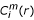(r) = { Number of x(j) such that d [x(i), x(j)] ≤ }/(N-m+1).

ApEn (N,r,m) is then defined as follows in equation (3)

ApEn = [ApEn m (N,r,m) - ApEn m+1(N,r,m + 1)]     (3)

The criterion of similarity, r, was chosen such that it was larger than most of the noise but at the same time not so large that detailed information about the system dynamics would be lost. On the basis of the work of Pincus et al , for m = 2, N = 1000 and r = 15% of the standard deviation of the data would produce reasonable statistical validity of ApEn.

In our study we use data set of 1000 adjacent R-R intervals. We divide the data set into smaller sets of length m = 2. This amounts to 500 smaller sub-sets. The next step is to determine the number of sub-sets that are within the criterion of similarity r = 15% of deviation of 1000 R-R intervals. Then we repeat the same process for the second sub-set till each sub-set is compared with the rest of the data set. This process computes the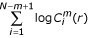part of equation (2) and N-m+1 = 1000-2+1 = 999. We repeat the same process for m = 3. Approximate entropy is then calculated using equation (3).

### Largest Lyapunov Exponent (LLE)

Lyapunov Exponent (λ) is a quantitative measure of the sensitive dependence on the initial conditions. It defines the average rate of divergence of two neighboring trajectories. An exponential divergence of initially nearby trajectories in phase space coupled with folding of trajectories, to ensure that the solutions will remain finite, is the general mechanism for generating deterministic randomness and unpredictability. Therefore, the existence of a positive λ for almost all initial conditions in a bounded dynamical system is widely used definition of deterministic chaos. To discriminate between chaotic dynamics and periodic signals Lyapunov Exponent (λ) are often used. It is a measure of the rate at which the trajectories separate one from other. The trajectories of chaotic signals in phase space follow typical patterns. Closely spaced trajectories converge and diverge exponentially, relative to each other. For dynamical systems, sensitivity to initial conditions is quantified by the Lyapunov Exponent (λ). They characterize the average rate of divergence of these neighboring trajectories. A negative exponent implies that the orbits approach a common fixed point. A zero exponent means the orbits maintain their relative positions; they are on a stable attractor. Finally, a positive exponent implies the orbits are on a chaotic attractor.

The algorithm proposed by Wolf et al  is used to Largest LE (LLE) from EEG data. For a given the time series x(t) for m dimensional phase space with delay coordinate t, that is a point on the attractor is given by

{ x(t), x(t + t),..., x(t + (m-1)t }

We locate nearest neighbor to initial point

{ x(t0), x(t0+ t),..., x(t0 + (m-1)t }

And denote the distance between these two points as L(t0). At a later time t1, initial length will evolve to length L'(t1). The mean exponential rate of divergence of two initially close orbits is characterized by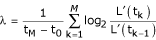In implementation of this program, the following set of numerical parameters has to be chosen:

P = { m, t, T, Smax, Smin,thmax}

where m is the embedding dimension, t is delay, T being evaluation time (= t k+1 - t k-1) and Smax, Smin are the maximum and minimum separations of replacement point respectively and thmax is the maximum orientation error. According to Das et al [Das et al, 2002] an embedding dimension between 5 to 20 and a delay of 1 should be chosen when calculating LE for EEG data. In our analysis we have chosen an embedding dimension of 10 and delay of 1.

### Detrended Fluctuation Analysis (DFA)

The concept of fractal is most often associated with geometrical objects satisfying two criteria: Self-similarity means that an object is composed of sub-units on multiple levels that statistically resemble the structure of the whole object. The second criterion for a fractal object is that it has a fractal dimension, also called fractal, that can be defined to be any curve or surface that is independent of scale. This concept of fractal structure can be extended to the analysis of physiological signals.

The DFA was used to quantify the fractal scaling properties of heart rate signals of short interval. Therefore, we apply DFA to the analysis of biological data, which calculates the root-mean-square fluctuation of integrated and detrended time series, permits the detection of intrinsic self-similarity embedded in a non-stationary time series, and also avoids the spurious detection of apparent self-similarity . This method has been applied to a wide range of simulated and physiologic time series in recent years [19, 8, 9, 21, 22].

To illustrate the DFA algorithm, the total length of the heart rate signal (N) is integrated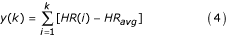Where HR(i) is the i th heart rate signal and HR avg is the average heart rate of N samples. Next, the integrated time series is divided into boxes of equal length n, a least-squares line is fit to the data (representing the trend in that box). The y-coordinate of the straight line segments is denoted by y n (k). Then, we detrend the heart rate data y(k), by subtracting the local trend, y n (k), in each box. The root mean square fluctuation of this integrated and detrended heart rate data is calculated by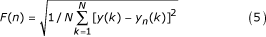This computation is repeated over all the box sizes to provide a relationship between F(n), the average fluctuation as a function of box size n.

In this study, the box size is ranged from 4 to ~300 beats. A box size larger than 300 beats would give a less accurate fluctuation value because of finite length effects of data.

Typically, F(n) will increase with box size. A linear relationship on a double-log graph indicates the presence of scaling i.e. F(n) ≈ nα. Under such conditions, the fluctuations can be characterized by a scaling exponent α, the slope of the line relating log F(n) to log(n). An α of 0.5 corresponds to white noise, α = 1 represents 1/f noise and α = 1.5 indicates Brownian noise or random walk. A good linear fit of the log F(n) to log(n) plot (DFA plot) indicates F(n) is proportional to nα, where α is the single exponent describing the correlation properties of the entire range of heart rate data [shown in Fig. 1]. However in some cases, we found that the DFA plot was not strictly linear but rather consisted of two distinct regions of different slopes separated at a break point n bp . This observation suggests there is a short range scaling exponent, α s , over periods of 4 to 13 [n bp ] beats, and a long – range exponent, α l , over long periods [Woo et al, 1992; Kamen et al, 1996].

## Results

Table 2 Variation of linear parameters for various age group normal subjects

Table 3 Variation of parameter for various age group normal subjects in the frequency domain

Table 4 Variation of nonlinear parameters for various age group normal subjects

The results of the nonlinear parameters for four different age group is presented in table 2. To test for a statistical significance of difference in original SD1/SD2, ApEn, LLE, α s , α l and the surrogate data, surrogate data series were generated to match each original signal. Then it is subjected to Student t test distribution. We found that, the surrogate data for SD1/SD2, ApEn, LLE, α s , α l and their original data are distinct and they differ by more than 50%. This rejects the null hypothesis and hence the original data contain nonlinear features.

### Surrogate Data

The purpose of surrogate data is to test for any nonlinearity in the original data . To test if the attractor geometry and correlation dimension are truly due to chaotic dynamics one must examine these characteristics for surrogate data sets.

Nonlinear indexes such as ApEn are computed for several surrogate data series. Their values are compared with that assumed by the nonlinear index computed for the original index . The demonstration of statistically significant difference in ApEn between the original and surrogate data are in keeping with the presence of nonlinear dynamics in the original data.

Surrogate data have Fourier decomposition with the same amplitudes as the empirical data decomposition but with random phase components. This is obtained from the Chaos Data Analyzer.

To test for a statistical significance of difference in original ApEn and the surrogate data, 10 surrogate data series were generated to match each original signal. Then it is subjected to Student t test distribution. We found that, the surrogate data ApEn and original data ApEn, are distinct and they differ by more than 50%. This rejects the null hypothesis and hence the original data contain nonlinear features.

Table 5 Results of various parameters using surrogate data

## Discussion

Nikhil Iyengar et al, have applied DFA to the normal heart rate variability signal . Recently, Echeverria et al, have applied detrended fluctuation analysis to the normal and Congestive Heart Failure (CHF) subjects. In this work, we have evaluated parameters α l , α s , ApEn and SD2 of the Poincare plots for the analysis of various cardiac abnormalities. And the results of these were subjected to't' test with more than 89% confidence interval giving excellent 'p' values in all cases.

The importance of ApEn lies in the fact that it is measure of the disorder in the heart rate signal. For the more regular and predictable heart rate values like complete heart block and ischemic/dilated cardiomyopathy type of abnormalities, the lower is the ApEn. On the other hand for the normal subjects where the heart rate is more random, ApEn has higher value. Hence the ApEn is used to find the unpredictability of fluctuations in the heart rate signals. This value decreases for the abnormal cardiac beats.

The pattern of Poincare plots of HRV data, its position and ranges of SD2 values are unique for particular type of cardiac abnormality. In this plot for the periodic data and for the normal heart rate signals the repeated stretching and folding of the trajectories, causes near by points to separate. Hence the position of the plot, its shape, SD2 computed with the HRV data may be considered as a characteristic parameter of diagnostic importance in clinical cardiology. For the normal subjects, shape of the plot is an ellipse and lies at the center of the quadrant and this position and shape of the plot shifts depending of the abnormality (Fig. 2-7). The value of SD2 indicates the long term variability. This value is incomparable with the SD1 in the case of abnormal cases.

The DFA technique is used to quantify the fractal scaling properties of short heart rate signals. This technique is a modification of root-mean-square analysis of random walks applied to non stationary data. From the root-mean-square fluctuation of an integrated and detrended time series is measured at different windows two parameters (α l and α s ) derived. These parts have different range of values for various types of cardiac abnormalities (as indicated by very low 'p' value as shown in table 2). The short time correlation exists for abnormal signals with varying degrees and this correlation is less for normal subjects and for normal subject the long term correlation is more. In the normal case the short range correlation is less and long range correlation is more. As the signal becomes abnormal this short range correlation becomes more.

SD1/SD2 shows the ratio of short interval variation to the long interval variation. This ratio is more in the case of child and old male subjects, indicating lesser R-R variability in the small interval. This ratio is less in the middle aged subjects, indicating higher R-R variability.

The importance of ApEn lies in the fact that it is measure of the disorder in the heart rate signal. It is a measure, quantifying the regularity and complexity of time series. It has higher value in the case of children and young and this value decreases as the subject grows old. Hence, the ApEn will have smaller value for middle and old aged subjects, indicating smaller variability in the beat to beat.

Largest Lyapunov exponent's (LLE) quantify sensitivity of the system to initial conditions and gives a measure of predictability. This value decreases with the aging indicating that the heart rate variability becomes less chaotic as the healthy subject grows old.

Short range correlation α s value is low in the abnormal signals. Similarly, the long range correlation α l values are more for the normal signal indicating the fractalness in the data and this range decreases as the subject grows old.

## Conclusion

Heart rate variability (HRV) signal can be used as a reliable indicator of state of the heart. It becomes less random with the aging(less chaotic). This is evaluated by using time domain, frequency domain and nonlinear parameters SD1/SD2, ApEn, LLE α s and α l . Different ranges of non-linear parameters for various age groups are presented with 'p' value ≤ 0.12.

## References

1. Buldyrev SV, Goldberger AL, Havlin S, Peng CK, Stanley HE, Simons M: Fractal landscapes and molecular evolution: modeling the myosin heavy chain gene family. Biophysical Journal 1993, 65: 2673–2679.

2. Cysarz D, Bettermann H, van leeuwen P: Entropies of short binary sequences in heart period dynamics. American Journal of Physiology-Heart and Circulatory Physiology 2000, 278: H2163-H2172.

3. Dahlberg ST: Gender difference in the risk factors for sudden cardiac death. Cardiology 1990, 77: 31–40.

4. Das A, Das P, Roy AB: Applicability of Lyapunov Exponent in EEG Data Analysis. Complexity International 2002, 9: 1–8.

5. Nagy Emese, Orvos Hajnalka, Bardos Gyorgy, Molnar Peter: Gender-related heart rate differences in human neonates. Pediatric Research 2000,47(6):778–780.

6. Galeev AR, Igisheva LN, Kazin EM: Heart rate variability in healthy six-to-sixteen -year- old children. Fiziol Cheloveka 2002,28(4):54–58. 10.1023/A:1016529931519

7. Grassberger P, Procassia I: Measuring the strangeness of a strange attractor. Physica D 1983, 9: 189–208. 10.1016/0167-2789(83)90298-1

8. Hausdorff JM, Purdon P, Peng C-K, Ladin Z, Wei JY, Goldberger AL: Fractal dynamics of human gait: stability of long-range correlations in stride interval fluctuations. Journal of Applied Physiology 1996, 80: 1448–1457.

9. Hausdorff JM, Peng C-K, Ladin Z, Wei JY, Goldberger AL: Is walking a random walk? Evidence for long-range correlations in the stride interval of human gait. Journal of Applied Physiology 1995, 78: 349–358.

10. Bonnemeier Hendrik, Uwe WiegandKH, Brandes Axel, Kluge Nina, Hugo KatusA, Richardt Gert, Potratz Jurgen: Circadian profile of cardiac autonomic nervous modulation in healthy subjects: Differing Effects of aging and Gender on heart rate variability. Journal of Cardiovascular Electrophysiology 2003,14(8):791–199. 10.1046/j.1540-8167.2003.03078.x

11. Huikuri HV, Makikallio TH, Airaksinen KEJ, Seppanen T, Puukka P, Raiha IJ, Sourander LB: Power-law relationship of heart rate variability as a predictor of mortality in the elderly. Circulation 1998, 97: 2031–2036.

12. Pan Jiapu, Willis TompkinsJ: Real Time QRS Detector algorithm. IEEE Transactions on Biomedical Engineering 1985,32(3):230–23.

13. Kamen PW, Krum H, Tonkin AM: Poincare plot of heart rate variability allows quantitative display of parasympathetic nervous activity. Clinical science 1996, 91: 201–208.

14. Kantz H, Kurths J, Mayer-Kress G: Nonlinear Analysis of Physiological Data. 1 Edition Berlin: Springer 1998.

15. Kevin DavyP, Christoper DesouzaA, Pamela JonesP, Douglas SealsR: Elevated heart rate variability in physically active young and older adult women. Clinical Science 1998, 94: 579–584.

16. Kobayashi M, Musha T: 1/f fluctuations of heart beat period. IEEE transactions on Biomedical Engineering 1982,29(6):456–457.

17. Malik M: Heart rate variability. Current Opinion in Cardiology 1998, 13: 36–44.

18. Iyengar Nikhil, Peng CK, AL Goldberger, La Lipsitz: Age-related alterations in the fractal scaling of cardiac interbeat interval dynamics. Am J Physiol 1996, 271: 1078–1084.

19. Ossadnik SM, Buldyrev SV, Goldberger AL, Havlin S, Mantegna RN, Peng CK, Simons M, Stanley HE: Correlation approach to identify coding regions in DNA sequences. Biophysical Journal 1994, 67: 64–70.

20. Peng CK, Buldyrev SV, Goldberger AL, Halvin S, Sciortino F, Simons M, Stanley HE: Long range correlations in nucleotide sequences. Nature 1992, 356: 168–170. 10.1038/356168a0

21. Peng CK, Halvin S, Stanley HE, Goldberger AL: Quantification of scaling exponents and crossover phenomena in nonstationary heartbeat time series. Chaos 1992, 5: 82–87. 10.1063/1.166141

22. Peng CK, Havlin S, Hausdorf JM, Mietus JE, Stanley HE, Goldberger AL: Fractal mechanisms and heart rate dynamics. Journal on Electrocardiology 1996,28(suppl):59–64.

23. Persson PB, Wagner CD: General principles of chaotic dynamics. Cardiovascular Research 1996, 31: 332–341. 10.1016/0008-6363(96)00006-5

24. Pincus SM: Approximate entropy as a measure of system complexity. Proc Nat Acad Sci USA 1991, 88: 2297–2301.

25. Pincus SM, Goldberger AL: Physiological time-series analysis: what does regularity quantify? Am J Physiol 1994, 266: 1643–1656.

26. Pincus SM, Viscarello RR: Approximate entropy: a regularity measure for fetal heart rate analysis. Obstetrics Gynecology 1992, 79: 249–255.

27. Ramaekers D, Ector H, Aubert AE, Rubens A, van de Werf F: Heart rate variability and heart rate in healthy volunteers: Is the female autonomous nervous system cardioprotective? Eur Heart J 1998, 19: 1334–1341. 10.1053/euhj.1998.1084

28. Rosenstien M, Colins JJ, De Luca CJ: A practical method for calculating largest Lyapunov exponents from small data sets. Physica D 1993, 65: 117–134.

29. Ryan SM, Goldberger AL, Pincus SM, Mietus J, Lipsitz LA: Gender-and age-related differences in heart rate: are women more complex than men? Journal of American College of Cardiology 1994,24(7):1700–1707.

30. Task Force of the European Society of Cardiology and North American Society of Pacing and electrophysiology: Heart Rate Variability: Standards of measurement, physiological interpretation and clinical use",. European Heart Journal 1996, 17: 354–381.

31. Terry KuoBJ, Lin Tsann, Cheryl YangCH, Li Chia-Lin, Chen Chieh-Fu, Chou Pesus: Effect of aging on gender differences in neural control of heart rate. Am J Physiol 1999, 46: H2233-H2239.

32. Theiler J, Eubank S, Longtin A, Galdrikian B, Farmer J: Testing for nonlinearity in time series: the method of surrogate data. Physica D 1992, 58: 77–94. 10.1016/0167-2789(92)90102-S

33. Tulppo MP, Makikallio TH, Takala TES, Seppanen T, Huikuri HV: Quantitative beat-to-beat analysis of heart rate dynamics during exercise. American Journal of Physiology 1996, 271: H244-H252.

34. Wilson PW, Evans JC: Coronary artery prediction. American Journal of Hypertens 1993,6(11pt 2):309S-313S.

35. Wolf A, Swift JB, Swinney LH, Vastano JA: Determining Lyapunov exponent from a time series. Physica D 1985, 16: 285–317. 10.1016/0167-2789(85)90011-9

36. Woo MA, Stevenson WG, Moser DK, Trelease RB, Harper RH: Patterns of beat-to-beat heart rate variability in advanced heart failure. American Heart Journal 1992, 123: 704–7. 10.1016/0002-8703(92)90510-3

37. Yamasaki Y, Kodama M, Matsuhisa M: Diurnal heart rate variability in healthy subjects: effects of aging and sex differences. Am J Physiol 1996, 271: 303–310.

## Author information

Authors

### Corresponding author

Correspondence to Rajendra Acharya U.

### Authors' Contributions

OWS and LYP carried out the data collection and software implementation. RAU, KN and CT carried out the design, analysis and coordination of the work. All authors read and approved the final manuscript.

## Authors’ original submitted files for images

Below are the links to the authors’ original submitted files for images.

## Rights and permissions

Reprints and Permissions

Acharya U, R., N, K., Sing, O.W. et al. Heart rate analysis in normal subjects of various age groups. BioMed Eng OnLine 3, 24 (2004). https://doi.org/10.1186/1475-925X-3-24